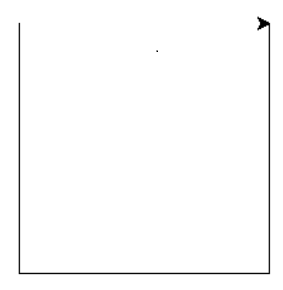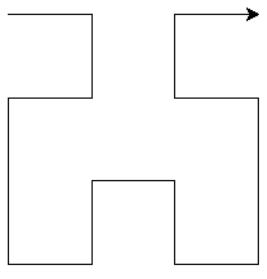# Python – Hilbert Curve using turtle

Fractal is a curve or a figure which repeats itself. It comprises a recursive pattern that repeats itself up to a desired level of nesting. Turtle graphics are provided in the turtle module which is used for drawing various shapes and patterns in Python.

A Hilbert curve is a curve which is formed by connecting a sequence of U-shaped curves arranged and oriented in different directions. These U-shaped curves are placed at a certain step size distance apart.

Let us examine a Level-1 Hilbert Curve. The following steps will draw a simple U curve.

Let y = 90 degree

1. Rotate y degree towards the right
2. Move step size
3. Rotate y degree towards the left
4. Move step size
5. Rotate y degree towards the left
6. Move step size
7. Rotate y degree towards the rightLet us examine and try to understand the level-2 Hilbert Curve.

Again, we assume that the turtle pointer points towards right initially. The following steps may be used to draw the curve:

1. Rotate 90 degrees towards the right
2. Create a hilbert curve at level 1 rotated by -y degrees (ie, y degrees in anticlockwise direction)
3. Move step size
4. Rotate y degrees towards the right
5. Create a level 1 hilbert curve rotated by y degrees (ie, y degrees in clockwise direction)
6. Rotate y degrees towards the left.
7. Move step size
8. Create a level 1 hilbert curve rotated by -y degrees
9. Rotate y degrees towards the right`turtle` methods used in this section are as follows :

• forward(): Used for moving the turtle forward by a given distance in the direction of the turtle.
• backward(): Used for moving the turtle backward by a given distance in the direction of the turtle.
• left(): Used for rotating the turtle in the left direction by a specified angle.
• right(): Used for rotating the turtle in the right direction by a specified angle.
• goto(): Used for moving the turtle to the location specified ((x, y) coordinates).
• penup(): Used for specifying that no drawing will be made while moving.
• pendown(): Used for specifying that that drawing will be made while moving.
• done(): Used to specify that the turtle work is completed.
Code for Hilbert Curve is given below :

 `from` `turtle ``import` `*`  ` `  `def` `hilbert(level, angle, step): ` ` `  `    ``# Input Parameters are numeric ` `    ``# Return Value: None ` `    ``if` `level ``=``=` `0``: ` `        ``return` ` `  `    ``right(angle) ` `    ``hilbert(level``-``1``, ``-``angle, step) ` ` `  `    ``forward(step) ` `    ``left(angle) ` `    ``hilbert(level``-``1``, angle, step) ` ` `  `    ``forward(step) ` `    ``hilbert(level``-``1``, angle, step) ` ` `  `    ``left(angle) ` `    ``forward(step) ` `    ``hilbert(level``-``1``, ``-``angle, step) ` `    ``right(angle) ` ` `  `def` `main(): ` `    ``level ``=` `int``(``input``()) ` `    ``size ``=` `200` `    ``penup() ` `    ``goto(``-``size ``/` `2.0``, size ``/` `2.0``) ` `    ``pendown() ` `    `  `    ``# For positioning turtle ` `    ``hilbert(level, ``90``, size``/``(``2``*``*``level``-``1``))        ` `    ``done() ` ` `  `if` `__name__``=``=``'__main__'``: ` `    ``main() `

My Personal Notes arrow_drop_upCheck out this Author's contributed articles.

If you like GeeksforGeeks and would like to contribute, you can also write an article using contribute.geeksforgeeks.org or mail your article to contribute@geeksforgeeks.org. See your article appearing on the GeeksforGeeks main page and help other Geeks.

Please Improve this article if you find anything incorrect by clicking on the "Improve Article" button below.

Article Tags :

Be the First to upvote.

Please write to us at contribute@geeksforgeeks.org to report any issue with the above content.